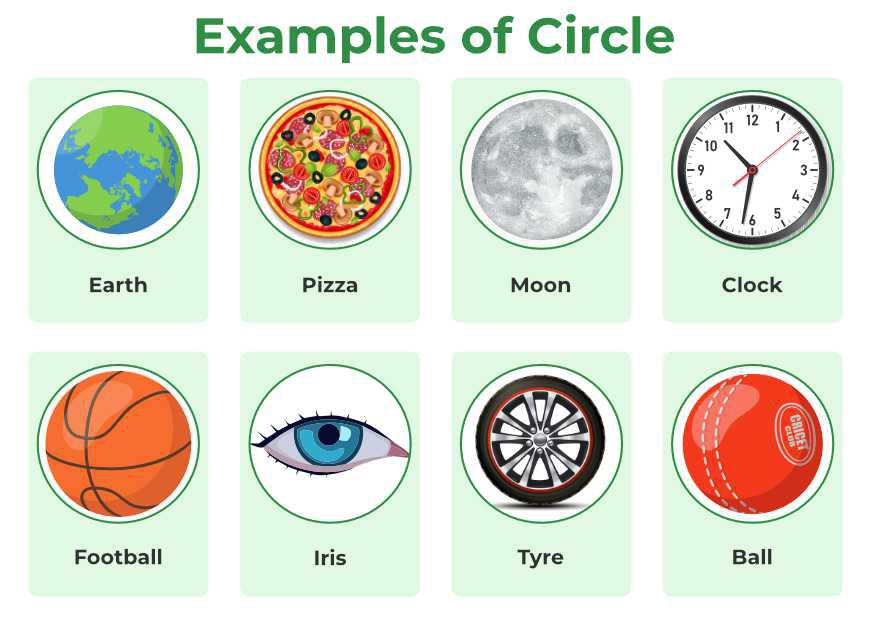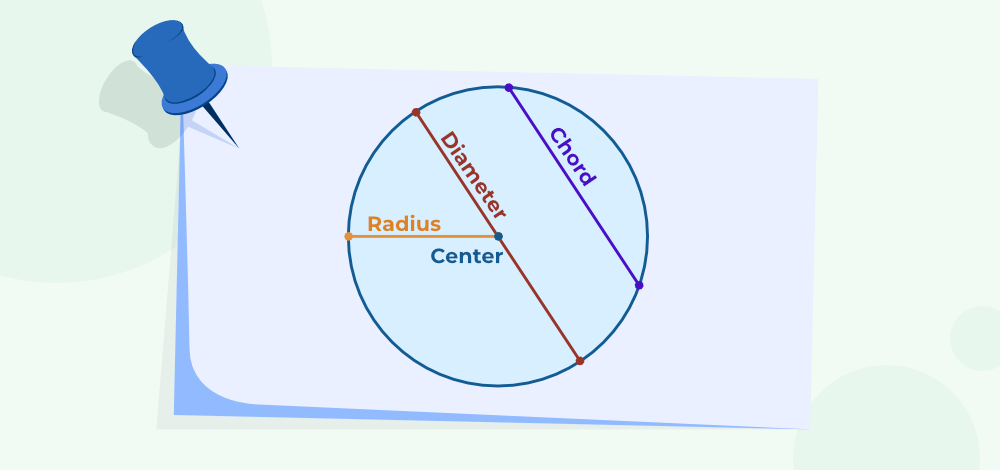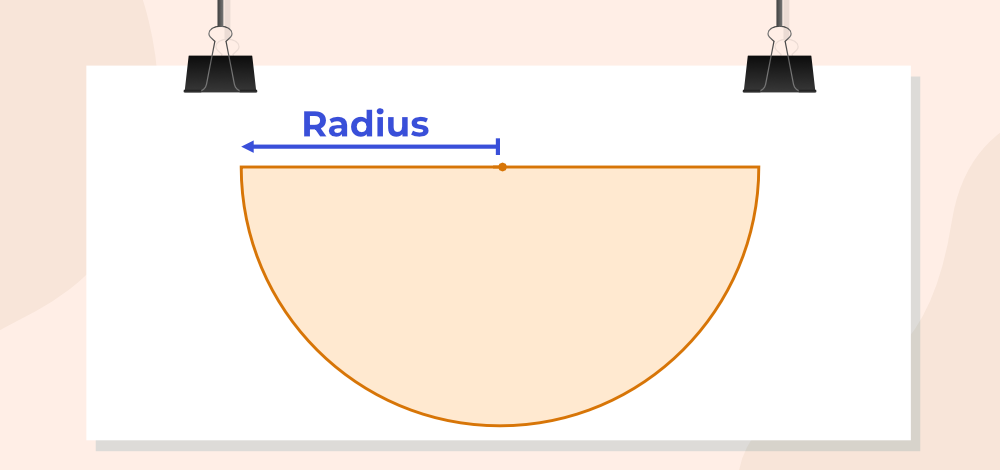# Circumference of a Circle – Definition, Formula, Examples, Facts

Circumference of Circle is defined as the boundary or the outer path covered by any circle. Generally, the Circumference of Circle is also called perimeter of circle, which is defined as the sum of all the sides of any figure, or the length of string that is required to cover the boundary of a circle. As commonly understood, a circle’s fundamental attributes include its perimeter and area of circle. This article will delve into the concept of the Circumference of a circle, also referred to as its Perimeter of circle, providing a comprehensive understanding of its definition, formula, and various approaches to calculating the circle’s perimeter, supported by numerous illustrative examples.

Circumference of circle and the Area of a circle are the most important formulas to study in the circle as the circle is the most fundamental shape in the evolution of mankind. The most important geometric measurements related to the circle are Circumference of circle, area of circle, circumference of a semi-circle, and area of a semi-circle and their formulas.Circle

## What is the Circumference of a Circle?

The perimeter of a circle is a curve that runs along the edge of the circle. The perimeter of a circle is also called the circumference of a circle. Circumference of circle is indicated by the letter C. It is slightly larger than three times its diameter. This is π of the diameter of the circle. The circumference is measured in cm, m, or Km. Conversion between these units can be done through various formulas like Centimeter to Meter Conversion Formula.

The numerical approximation of pi is 3.1415926535897…, denoted by the Greek letter π (pronounced as “Pi”). Pi is an incessant, non-terminating value.Circumference of Circle

## Definition of Circumference of Circle

The Length of the Boundary of the Circle is called the Circumference of the circle or Perimeter of the circle. In contrast, the area of circle delineates the space it occupies. Let’s say we convert the circle into a straight rod. The length of the rod is basically the circumference of the circle.

When employing the formula to compute a circle’s circumference, the radius of the circle becomes a critical factor in the calculation. Consequently, it is imperative to possess either the radius or diameter value in order to determine the circle’s perimeter.

## How to Find Circumference?

Approach 1: Due to its curved nature, directly measuring the length of a circle with a ruler or scale isn’t feasible as it is for polygons like squares, triangles, and rectangles. However, you can determine the circumference of a circle using a thread. By tracing the circle’s path with the thread and marking points along it, you can measure this length using a standard ruler.

Approach 2: A precise method to ascertain a circle’s circumference involves calculation. To do this, the circle’s radius must be known. The radius of a circle is the distance from its center to any point on its circumference. In the illustration below, a circle with radius R and center O is depicted. The diameter, twice the radius of the circle, is also shown.

### Center

A fixed point related to a circle; such that all the points on the boundary of the circle are at an equal distance from that fixed point, is called the center of a circle.

Radius is the minimum distance between the center and any point on the circumference. As we only need a fixed point, center, and radius to construct a circle so the radius is the most fundament aspect of the circle without it we can’t define the circle.Circle

### Chord

A line joining any two points on the circumference of a circle is known as a chord.

### Diameter

A chord of the circle which passes through the center is known as the Diameter. Also, the diameter is the longest chord of the circle.

## How to Find the Circumference of a Circle?

The circumference of a circle is equal to the length of its boundary, but the circle is a curved shape, its circumference can’t be measured with a ruler. The correct way to find the perimeter of a circle is to calculate it using the formula C = 2π × r. If the diameter or radius of a circle is known circumference of the circle can be easily calculated.Circumference of circle

## Formula for Circumference of a Circle

### Circumference to Diameter

The ratio of Circumference to the Diameter of a circle is always a constant and that is π. Using this fact we can calculate the formula for circumference.

Circumference/Diameter = π

or

Circumference = π×Diameter

As the Diameter of a circle is two times the circle’s radius, the ratio of Circumference to the radius of a circle is also always a constant, which is 2π. Using this fact we can also calculate the formula for the circumference.

or

## What is the Circumference to Diameter Ratio?

The ratio of the circumference to the diameter of any circle is a constant known as pi (π). Denoted by π, this value remains consistent for all circles, irrespective of their size. The relationship is expressed as:For all circles, big or small, this ratio stays the same. The approximate value of π is commonly taken as 3.14 or 22/7.

## Area of Circle Formula

Area enclosed by the circumference of a circle is known as the Area of a Circle. In other words, all the area inside the boundary of the circle is considered its area and it is calculated using the formula

A=πr2

Where r is the radius of the circle and

π is the constant with an approximate value of 22/7 or 3.14.

## Difference between Area and Circumference

Area: It is basically defined as the measure of square units inside the circle.

Circumference: It is basically defined as length around the circle.

## Circumference of a Semicircle FormulaSemi – circle

If a circle is split into two equal parts it is called a semi-circle. The circumference of a Circle is defined as the overall length of its boundary, which is given by

Circumference of Semi-Circle = πr + d

where,
r is the radius of the circle,
d is the diameter of the circle.

## Area of a Semicircle Formula

Area of a semi-circle is calculated by taking half of the area of the circle. i.e. the area of a semi-circle is ½ × the area of a circle. the formula for the area of semi circle is given as

Area of semi-circle =  ½ × πr2

where r is the radius of the circle.

## Solved Examples on Circumference of Circle

Example 1: What is the circumference of a circle with a diameter of 2 cm?

Solution:

Given, Diameter = 2 cm

By using formula of circumference of a circle,

C = π × d

Where, C = Circumference, π (pi) = 3.14 and d = diameter

C = 3.14 × 2

C = 6.28 cm.

Example 2: What is the circumference of a circle with a radius of 3 cm?

Solution:

By using formula of circumference of a circle,

C = 2 × π × r

Where, C = Circumference, π (pi) = 3.14 and r = radius

C = 2 × 3.14 × 3

C = 18.84 cm.

Example 3: What is the circumference of a circle with a diameter of 14cm?

Solution:

Given, Diameter = 14 cm

Using the formula of circumference of a circle,

C = π × d

Where, C = Circumference, π = 3.14 and d = 14 cm

C = 3.14 × 14

C = 43.96 cm.

Example 4: What is the circumference of a circle with a radius of 10 cm?

Solution:

Using the formula of circumference of a circle,

C = π × 2r

Where, C = Circumference, π = 3.14 and r = 10 cm

C = 3.14 × 2(10)

C = 62.8 cm.

Example 5: What is the circumference of a circle with a radius of 25 cm?

Using the formula of circumference of a circle,

C = 2 × π × r

Where, C = Circumference, π = 3.14 and r = 25 cm

C = 2 × 3.14 × 25

C = 157 cm.

Example 6: What is the circumference of a circle with a radius of 7.5 cm?

Using the formula of circumference of a circle,

C = 2 × π × r

Where, C = Circumference, π = 3.14 and r = 7.5 cm

C = 2 × 3.14 × 7.5

C = 47.1 cm.

Also Check:

## FAQs on Circumference of Circle

### 1. What is the Circumference of a Circle?

The perimeter of a circle is a curve that runs along the edge of the circle. In other words, the boundary of the circle is its circumference.

### 2. What is the value of π(pi)?

For general purpose calculations, we use the approximate value of π, which is 22/7 or 3.14, but as π is an irrational number, it has infinitely many digits in its expansion. The value of for the first 10 digits in the expansion after the decimal, is 3.1415926535. . .

### 3. What is the difference between perimeter and circumference?

One and only difference between perimeter and circumference is that perimeter is a general word for all polygons and curves, while circumference is only used for the circle. In other words, we can calculate the perimeter of a circle, but we can’t calculate the circumference of a square.

### 4. How to Calculate Diameter from Circumference?

The formula for circumference = diameter × π

Or, diameter = circumference/π

So, the diameter of the circle in terms of circumference will be equal to the ratio of the circumference of the circle and π.

### 5. What are the steps involved in finding the circumference of a circle if its area is given?

Step 1: Find the radius using the area of the circle formula (A = πr2).
Step 2: Now, substitute the radius value in the circumference formula (C = 2πr) to get the answer.

### 6. Which formula is used to find the perimeter of a circle, when its area is given?

If the area of a circle is given, then the formula to calculate the perimeter or circumference of a circle is given by: C = 2√(πA) units.

### 7. What is the circumference of a semi-circle?

The formula for the circumference of a semicircle is C = (πr + 2r), where r is the radius. We know that diameter is twice the value of the radius, d = 2r.

The formula for the circumference of a semicircle becomes C = π(d/2) + d.

### 8. What is the circumference of a quadrant of a circle?

The quadrant of a circle is the sector of the circle whose sectorial angle is 90°. The perimeter of the quadrant of a circle is one-fourth of the circumference and 2 times the radius of a circle.

### 9. What is the Circumference formula?

Circumference formula for circle = Circumference = 2π×Radius

Circumference formula for semi-circle = πr + d

### 10. Find the circumference of a circle in terms of π, if the radius measures 3 cm.

As established, we recognize that the circumference equals 2πr, which in this case is 2π(3) = 6π. Hence, if the radius of a circle measures 3 cm, its circumference would amount to 6π cm.

### 11. How To Calculate The Circumference of a Circle?

To calculate the circumference of a circle, you can use the formula:

Circumference =where:is a mathematical constant approximately equal to 3.14159.is the radius of the circle.

Alternatively, you can use the diameter (d) instead of the radius with the formula:

Circumference} =Simply plug in the value for the radius or diameter into the formula, perform the multiplication, and you’ll have the circumference of the circle.

### 12. How do you find the circumference of a circle with a thread?

To find the circumference of a circle, you can use a simple method with a thread:

1. Wrap a thread around the circle, marking the initial and final points.

2. Measure the length of the thread with a ruler; this approximates the circumference.

For more accuracy, use the formulaor, as discussed in the next section.

Whether you're preparing for your first job interview or aiming to upskill in this ever-evolving tech landscape, GeeksforGeeks Courses are your key to success. We provide top-quality content at affordable prices, all geared towards accelerating your growth in a time-bound manner. Join the millions we've already empowered, and we're here to do the same for you. Don't miss out - check it out now!

Previous
Next